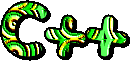## Monday, 11 June 2012

### IO manipulators

Q1: What is the purpose of iomanip.h header file?

Answer: The header file iomanip.h defines functions and operators to format input and output streams. These are used in conjunction with insertion (<<) and extraction (>>) stream objects.

Although C++ defines a number of functions, Turbo C++ define a set of these. e.g.
• numerical base formats: hex, oct, dec
• setw: sets the width of the field
• setprecision : sets the number of decimal places you want to display.
• setiosflags: you may enable display stream flags (ios::showbase, ios::uppercase, ios::showpos)
• resetiosflags: disable stream flags.

Q2: Write a program to display a number in hexadecimal, octal and decimal form.

Answer: The header iomanip.h defines hex, oct and dec format flags to display numbers in respective number systems. (e.g. 15 will become 0f in hexadecimal notation and 17 in octal system).
By default C++ uses decimal format to display numbers. To display numbers in uppercase (e.g. 0F instead of 0f), we use setiosflags(ios::uppercase) manipulator. ios::uppercase shows hexadecimal and scientific numbers in uppercase
Similarly, to display base format e.g. (hexadecimal uses 0x notation), use manipulator setiosflags(ios::showbase).

```#include <iostream.h>
#include <conio.h>
#include <manip.h>

int main()
{
clrscr();             // clear the screen
int x = 27;
cout << hex << "value of x in hexadecimal form:" << x << endl;
cout << oct << "value of x in octal form:" << x << endl;
cout << dec << "value of x in decimal:" << x << endl;
// show basefield notation, and in uppercase
cout << setiosflags(ios::showbase);
cout << setiosflags(ios::uppercase);
cout << hex << "value of x in hexadecimal:" << x << endl;
cout << oct << "value of x in octal form:" << x << endl;
cout << dec << "value of x in decimal:" << x << endl;

getch();
return 0;
}
```

The program will have the following output:

value of x in hexadecimal form:1b
value of x in octal form:33
value of x in decimal:27
value of x in hexadecimal:0X1B
value of x in octal form:033
value of x in decimal:27

Q3: An accountant is calculating profit and loss statement. He wants to show the numerical values prefixed by '+' sign if they represent profit and with '-' sign as loss indicator.  How can he show the formatted output? Write a C++ program to show this.

Answer: The function setiosflags(ios::showpos) in iomanip.h turns on + sign for positive numbers.
Similarly, one can use resetiosflags(ios::showpos) to turn it off. Following snippet shows:

```#include <iostream.h>
#include <conio.h>
#include <manip.h>

int main()
{
clrscr();             // clear the screen
int x = 27;
int p = 2, q = -4, r = 0;
float fval = 100.12;
cout << "p = " << p << " q = " << q << " r = " << r << endl;
cout << "fval = " << fval << endl;
cout << setiosflags(ios::showpos) << "\np = " << p << " q = " << q << " r = " << r << endl;
cout << "fval = " << fval << endl;
cout << resetiosflags(ios::showpos) << "\np = " << p << " q = " << q << " r = " << r << endl;
cout << "fval = " << fval << endl;

getch();
return 0;
}
```

Output on screen will be:
p = 2 q = -4 r = 0
fval = 100.12000

p = +2 q = -4 r = 0
fval = +100.12000

p = 2 q = -4 r = 0
fval = 100.12000
c:\tc\code>_

Q4: Write a C++ program to show how a number can be displayed as right or left justified. Also show how can we set the width of the displaying field.

Answer: iomanip.h provides more iostream flags to align output to left or right. ios::left flag aligns output to left, while ios::right aligns output to right. ios::internal justifies output internally.

The manipulator setw sets the field width of the output or input parameter.

By default, blankspace characters fills up the justification. You can change the fill-charatcer using cout.fill(char) function.

Following snippet shows the use of these manipulators:

```#include <iostream.h>
#include <conio.h>
#include <manip.h>

int main()
{
clrscr();             // clear the screen
cout << -123.45 << endl;
cout << setw(10) << -123.45 << endl;
cout << setw(10) << setiosflags(ios::left) << -123.45 << endl;
cout << setw(10) << setiosflags(ios::right) << -123.45 << endl;
cout << setw(10) << setiosflags(ios::internal) << -123.45 << endl;
cout.fill('*');
cout << setw(10) << 210.95 << endl;
cout << setw(10) << setiosflags(ios::left) << -210.45 << endl;
cout << setw(10) << setiosflags(ios::right) << -210.45 << endl;
cout << setw(10) << setiosflags(ios::internal) << -210.45 << endl;

getch();
return 0;
}
```

The output on screen will look like:
-123.45
-123.45
-123.45
-123.45
-   123.45
****210.95
-210.45***
***-210.45
-***210.45

Q5: A programmer is writing a module for a salary calculation package. After computing the salary, he wants to display paisas upto two decimal points. Write a c++ program to show how a floating number e.g. 12.578 is displayed as 12.57

Answer: C++ manipulators i.e. setf(ios::fixed), setf(ios::showpoint) and setprecision(int) be used here.
The format option ios::showpoint displays decimal point and trailing zeros for all pointing point numbers, even if decimal places are not need.
setprecision(2) ensures number of digits to be displayed after the decimal point.
The snippet is:

```#include <iostream.h>
#include <conio.h>
#include <manip.h>

int main()
{
clrscr();             // clear the screen
cout << 12.578 << endl;
cout.setf(ios::fixed); // display floating numbers NO scientific notation
cout.setf(ios::showpoint);
cout << setprecision(2) << 12.578 << endl;  // displays 12.57
cout << setprecision(2) << 12.5 << endl;   // displays 12.50
getch();
return 0;
}
```

Go to Snippets-4
.#### Post a Comment

We love to hear your thoughts about this post!